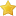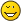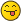• 0 Vote(s) - 0 Average
• 1
• 2
• 3
• 4
• 5
 Brainf**k Tutorial Author Message
IronsideSite OwnerPost: #1 Brainf**k Tutorial For some reason, when posting, A BUNCH of +/-'s were removed, leaving a lot of the code which produces output as unusable(the idea is the same). Keep this in mind, sorry. I will note the incorrect ones with an *. Also, the correct document is linked at the bottom. I haven't seen much of this around. Brainf**k(bf) is an esoteric(purposely confusing) language with only 8 commands. There are no variables, instead there is a 1D array which you can store data in, and consequently, read from. Despite all this it IS turing complete, a challenge, and extremely fun! There are various implementations, but I will be talking about a BF implementation with these rules: 30000 cells (elements in the array) 8-bit storage (256) Tape wrapping (1D array) On read, no data is affected Values are initialized to 0 and pointer is set on cell 0 A windows application known as BFdev will meet these requirements, along with the ability to read memory and step through actions. (I actually run Linux exclusively, but run it under wine.) Values are stored in 8-bit cells, each value corresponds to it's proper ASCII code, meaning 48 is 0, and 97 is the letter a. Most of the examples I will post here will not have output, meaning you will need to read the memory cells. Output is not hard to implement though, you simply offset the value, if it's a number, by 48, and 97 if it's a lowercase letter. I will first start with what the commands are... > - increment the data pointer (to point to the next cell to the right). < - decrement the data pointer (to point to the next cell to the left). + - increment (increase by one) the byte at the data pointer. - - decrement (decrease by one) the byte at the data pointer. . - output the value of the byte at the data pointer. , - accept one byte of input, storing its value in the byte at the data pointer. [ - if the byte at the data pointer is zero, then instead of moving the instruction pointer forward to the next command, jump it forward to the command after the matching ] command*. ] - if the byte at the data pointer is nonzero, then instead of moving the instruction pointer forward to the next command, jump it back to the command after the matching [ command*. --Wikipedia definitions Think of the "Tape" as something like this: Code: ```0]0]... -^``` ^ being the pointer, it is pointing to cell 0, farthest on the left...(unless you wrap around of course) Lets perform > Code: ```0]0]... ----^``` It moved to the second cell! (Called cell 1) The reverse would happen if < was performed. Let's + to the second cell. Code: ```0]0]... ----^``` It is now holding the value of 1! Consider this though, if you simply wrote + in a bf program, it would increment cell 0, so in order to get the results shown here, you would have to actually perform >+ But wait, if we reinitialize everything(reset) and decrement(-) look what happens! Code: ```0]0]... --^``` Why you ask? Because of underflow it will wrap around, the same can occur with pointer movement. Performing < on the first cell will bring you to the 30000th cell! Know this, cell values can hold 255 on an 8-bit BF system, more if it's 16 or 32-bit, but this is usually slower and unneeded. Also, 0-255 correspond with their ASCII values, ',' takes user input, which if it was 'a' would set the cell to 97, and if it was 0 the cell would contain 48! '.' prints out this data. If the cell contains 5, it will not print 5, it will more than likely print nothing as this is an invisible character in most cases. to print 5 out, the cell should contain 48 + 5, which is 63, or the ASCII value for 5. Now that you can reference what the commands mean, let's run through some easy snippets. Note: Anything that is not a brainf**k operator is considered a comment. First, I recommend you obtains BFdev, and when you run code, first open the Run submenu, and set click Watch Program Operation, then set running speed to a fairly slow level. (1-5hz) The values we see in these snippets will not be outputted, so we instead have to read the memory, which is not complex at all to do. Code: `[-]` Consider this snippet; the first bracket says to only enter the loop if the current cell is not equal to 0. So if it WAS greater than zero(overflows/underflows included) then it would decrement(-) the data by 1 in the current cell. It would then continue towards the second bracket, then inquiring if the cell data is still greater than 1, if so, repeat. In short, the snippet will clear a cell(set it to 0) and then stop. Code: `[[[-]>]` Now, reasoning that the previous snippet erases a cell, then this snippet must also erase a cell, but, this time it's inside a loop with a > initializing it. The action produced by this code would be, if the current cell contains data, erase it, then move to the next cell, and if that cell contains data, erase it and continue, It would clean data until it hit a cell with no data contained. basically sweeping up. Code: `+++[>+++<-]` What's this? A different snippet!? consider what this snippet is doing, it adds 3 to cell 0, then it enters a while loop([]), which first moves the pointer right one cell, and adds three to that cell. cell 1 now contains 3, and the pointer is then moved back to cell 0, where it subtracts 1 leaving it with 2. It then checks if the cell has data greater than 0 and since 2 is greater, it continues, as you can see, it will loop through 3 times, increments cell 1 3 times per loop. It's multiplying! 3 x 3! Here are some more examples: Code: ```++[<+++>-] ++[>---<-}  (subtracts instead, sound familiar?)``` How would we move data to a cell from another? To do so, you simple set the cell with the information as the counter(the cell which denotes how long to loop) and increment a cell inside the loop. Code: ```+++  cell 0 contains DEC 3  this is the counter [  initialize loop    -  subtract one from the counter    >+  move forward and add one to cell 1    <  move back and check the loop against the counte ]    recurse until counter is 0``` Now, if you noticed, we destroyed the original data in cell 0! How can we replace that? Well we need to use some scratch space(temporary for the function) Code: ```+++  cell 0 contains DEC 3  The counter [    initialize loop    -  subtract one from the counter    >+    add one to cell 1    >+    add one to cell 2    <<    subtract 1 from counter ]  rinse and repeat alright alright no rinsing``` But wait, the data is in cell 1 and 2, not 0 and 1! Well that is simple, just do a destructive copy(first one) from cell 2 to 0 using 2 as the counter. Now you may be asking, this is good and all, but I don't want to look at the memory the entire time! Well, have no fear, we can fix that! The idea is, if you are printing letters, offset the value to 97 and increment to get the desired lower case letter, do the same for numbers with 48. Code: ```<++[>++<-]>---   Hmm cell 0 now contains 97! What do we do now?? .  WHAT? One command? This is heresy! No ~seriously~ you just output the letter 'a' for the world to see!``` That's that, try to output hello! (BFdev has a text generator too, but it uses simple algorithms and they aren't very great, but they do work.) Alright, I get it, you understand, well how about some REAL CODE(If you don't understand, there are more simple defined examples under these. Note, most of these don't have stdout, because I was lazy, but the actual brain is written. Code: `,[>>>+<<<-]>,[>>+<<-]>>[<+>[-]]` Ponder it, keep pondering, moar, once you give up, open the spoiler...Try breaking it into pieces first, it may help. [spoiler]It performs the logical function OR! it takes 2 inputs and leaves the output on cell 0002. Search that memory![/spoiler] What? That was too hard???? How about my unbridled, crappy looking code! Code: `,[>+>+<<-]>>>,[>+>+<<-]<<[>>>[>>+<<-]>>-<<-]>>+  <[-]<[-]<[-]<[-]<[-]>>[<<<<+>>>>-]` The separated code is simply to clean up the mess I made and move the output to cell 0002. [spoiler]REALLY? It was easy, noob. Just kidding, that was an unfamiliar logic operation known as imply. The output is always 1 if input A is 0, and if A is 1, the output is only 1 when B is 1 as well. A implies B.[/spoiler] Here is a way to show output for these operations, demonstrated with XOR: Code: ```* [-]>[-]<, <<<<++[>>>>++<<<<-]>>>>. this should be 48 <<<<++[>>>>--<<<<-]>>>> this should match the above with the substituted symbol inside [>>>+<<<-]>, <<<<++[>>>>++<<<<-]>>>>. ditto <<<<++[>>>>--<<<<-]>>>> ditto [>>+<<-]>>[-[<->-]<+>] <<++[>++<-]>.``` Well, that's it for now, I might record a video of some code debugging, and I will post some more snippets later. More: BF compilers exist, and they work well, I recommend getting one, and compiling this following BF program, and then running it in cmd or terminal. Code: `Correct code will be linked at the bottom.` It's actually pretty simple, it prints out ^[[H^[[2J Which will prompt the shell to clearscreen, but a friend has suggested that ^[[2J should work as well. Let's do some real logic processing code I showed you OR, IMPLY, and XOR w/output, let's see the rest... When using these, and inputting, use the number, not text input, and only use 1 or 0, as they are binary operators. In case you didn't know, the same goes for the previous examples of logic operations as well. Most of the time, the output is placed in cell 0002. Code: `,[>,[>+<-]<-]` This is the most simple of them all! AND, if both are 1, then output is 1. Code: `,>+<[>-]` This negates the input, i.e. 1 is 0, 0 is 1. Code: `++[>,[>+<-]<-]>>-[-<]>+` This is not a good implementation and it can't be used in conjunction with other operations without some tests to change data slightly, but this is logical equivalence, i.e. x == y. The reason it is not great, is because one of the outputs produces 255, which, while it will test true like 1, it will offset other tests. One way to fix this, is to test if it can be subtracted, if it still contains data, then increment it three times. Disclaimer: These snippets(above) are not the most efficient way of doing things, but they get it done, don't follow me exactly and find your own style,. These next incorrect ones are fairly important to have correct, you should be able to see what the idea is. Alright, let's take a break from all that, let's have a look at this: Code: `*++++[>++ ++<-]++` The result will be 45, correct? Instead, lets perform it this way Code: `*++[>++ ++++<-]` It's the same, is it not? When you are writing this things, try to get close through the multiplication for 48, instead of 5*10-2, perform 4*12, 6*8, or even something more complex....Assuming it's shorter. Let's try.. 5*10-2 Code: `*>++[<++ ++>-]<--` 4*12 Code: `*>++++[<++ ++>-]` 6*8 Code: `*>++[<++++ ++++>-]` 4*3*4 Code: `*>++++[>+++[>++++<-]<-]` So, 4*3*4 seems to be the shortest, but is it the fastest? It's all up to you at this point, but it's cool to look at.